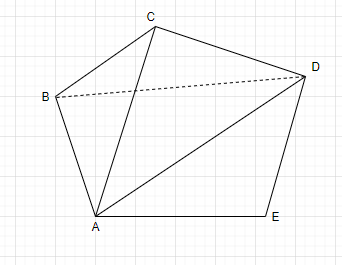QuestionAnswers

# In the given five sided closed figure, find $\angle$ EAB + $\angle$ ABC + $\angle$ BCD + $\angle$ CDE + $\angle$ DEA.$A$. ${520^ \circ }$   $B$. ${540^ \circ }$   $C$. ${530^ \circ }$   $D$. ${140^ \circ }$Verified
112.2K+ Views
Hint: Here we will proceed by taking three triangles from which the pentagon is made. Then by using the sum of all interior angles of a triangle i.e. ${180^ \circ }$, we will add all the angles and we will get the required sum of the angles.

$\vartriangle ABE \\ \vartriangle EBD \\ \vartriangle DBC \\$
Now we will use the property of the triangle i.e. sum of all interior angles of a triangle is ${180^ \circ }$.
The sum of all its angles$= 3 \times {180^ \circ } = {540^ \circ }$
So $\angle$ EAB + $\angle$ ABC + $\angle$ BCD + $\angle$ CDE + $\angle$ DEA = ${540^ \circ }$
$\therefore$ Option B is correct.
Note: There are also other ways by which we can solve this problem as if we use angle sum property of pentagon i.e. S = $\left( {n - 2} \right){180^ \circ }$where n is the number of sides of the pentagon. Then we will put the value of n and get the answer as ${540^ \circ }$.